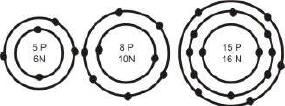# UP Board Important Questions for Class 9 Science

Science is a subject that requires analytical thinking & practical approach. Science consists of Physics, Chemistry & Biology. Mainly during the exam timing, students mostly search for UP Board Important Questions for Class 9 Science. And to get those papers, students generally purchase many local authors books & model sample papers. Solving from that can be useful as those model papers will contain the quality questions. Meanwhile, to help in your UP Board exam preparations we have provided the UP Board Important Questions for Class 9 Science.

### Highlights of UP Board Class 9 Science Important Questions

Practising important questions will help in your revision & make your learning effective. Here, are some of the features UP Board Important Questions for Class 9 Science:

• This set of Important UP Board Class 9 Science Questions will give you an idea of the type of different questions that might be asked in the exam.
• The questions are being prepared by the team of Experts.
• These Important Class 9 Science Questions of UP Board are based on the important topics of the syllabus.
• You will also find new types of questions.

## UP Board Important Questions for Class 9 Science

Here we have provided the set of Class 9 Important Questions of UP Board that consist of long answers i.e 3 or 5 marks.

The Questions number from 1 to 11 below carries 3 marks each:

Q1) List any three human activities which would lead to an increase in the carbon dioxide content of the air.

Q2) What information do you get from the figure given below about the atomic number, at the mass number and valency of atoms X, Y, and Z.Q3) Write the given statement in your answer books after filling in the blanks?

a) Pila and Unio have an external shell and belong to the phylum_____.

b) Free-living marine animals with water driven tube system are in the phylum ______.

c) To which phylum do sponges belong_____.

Q4) Differentiate between monocots and dicots. Give two differences and one example of each?

Q5) Define Sanyam and Svasthya? How are the two related?

Q6) (a) State the law of Constant Proportion.

(b) In a compound Carbon and Oxygen react in a ratio 3: 8 by mass to form carbon dioxide. What mass of oxygen is required to repeat to react completely with 9g Carbon?

Q7) (a) What are the conditions for work to be done?

(b) An electric bulb of 60W is lighted for 10 hours a day. What is the amount to be paid in a month of 30 days, if one unit of electricity of costs Rs. 3.50?

Q8) List any three ways of preventing the spread of air-borne diseases.

Q9) (i) State “Archimedes principle”.

(ii) The volume of 50g of a substance is 20 cm3. If the density of water is 1gm cm 3, will the substance float or sink?

Q10) (a) On which characteristics of sound wave do the following properties depend?

(i) loudness (ii) Pitch

(b) Calculate the time for which the sensation of sound persists in our brain if the minimum distance of the obstacle from the source of sound is 17.2m (speed of sound in air =344m /s)

Q11) (a) What is the causal organism for Swine flu?

(b) Suggest two measures that the local authorities of your neighborhood should take to bring down the incidence of diseases like malaria, typhoid, and dengue?

The Questions number from 12 to 20 below carries 5 marks each:

Q12) a) A child hers an echo from a cliff 4 seconds after the sound from a powerful cracker is produced. How far away is the cliff from the child? Speed of sound = (340 m/s).

b) Derive a relation between wavelength, frequency and wave velocity.

Q13) Draw a neatly labelled structure of the human ear and its working.

Q14) Give reasons for the following:

(a) Bryophytes are called “amphibians of the plant kingdom.”

(b) Spiders and scorpions are very different from each other but are placed in the same phylum –Arthropoda.

(c) Platyhelminths and Nematodes possess a Pseudocoelom. Write the name of the following:-

(i) Body is segmented

(ii) Reptile which has four chamber heart.

Q15) Write the main characteristics of phylum Porifera and Aves give one example of each with its diagram.

Q16) An Element “X” has 13protons, 13electrons and 14 neutrons.

a) What is its atomic number of “X‟?

b) Identify the element.

c) What is its valency? What is the number of valence electrons is “X”?

d) What is the type of ion formed by “X”? Why?

e) Name the scientists who discovered electrons and protons.

Q17) (a) Describe Bohr‟s model of an atom.

(b) Draw a sketch of Bohr‟s model of an atom with 3 shells.

(c) What was the drawback of Rutherford‟s model of an atom?

Q18) (a) Prove the law of conservation of energy for a stone moving vertically down.

(b) A boy of mass 50kg runs up a staircase of 45 steps in 9s. If the

height of each step is 15cm, find his power [g= 10 ms-2]

Q19) (a) Define the term „kinetic energy‟.

(b) Derive an expression for kinetic energy for an object of mass “m‟ moving with a velocity “v‟.

(c) A certain force acting on a 20 kg mass changes its velocity from 5m/s to 2 m/s. Calculate the work done by the force.

Q20) (a) What are the greenhouse gases?

(b) Give a diagrammatic representation of the Carbon Cycle in nature.

Alternatively, Class 10 students can also refer to the UP board 10th model paper downloaded from BYJU’S for more reference.

Did you find this page on “UP Board Important Questions for Class 9 Science” Useful? Stay tuned for more updates on UP Board Exam and other resources like 10th Class Science paper UP board.